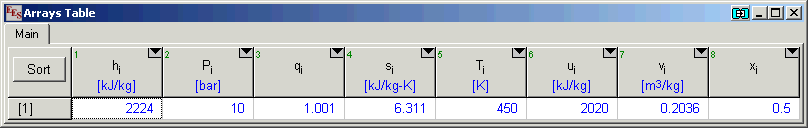Contents - Index

NH3H2O (Ammonia-Water)

NH3H2O provides the thermodynamic properties of ammonia-water mixtures in subcooled, saturated and superheated conditions.  Since NH3H2O is a mixture, its implementation differs somewhat from that for the pure substances.  First, it requires 3 independent properties to fix the state.  Second, there is a new property function called MassFraction that returns the mass fraction of ammonia.  Third, property designators X and Q are used for NH3H2O, which differs from their use for real fluids.  These properties  are identified by a single case-insensitive letter followed by an equal sign.  The property designators that are applicable to NH3H2O and their meanings are:

H=specific enthalpy

P=pressure

Q=quality (Saturated states, 0<=Q<=1; Subcooled. Q=-0.001; Superheated Q=1.001).

S=specific entropy

T=temperature

U=specific internal energy

V=specific volume (=1/density)

X=mass fraction (Note that X designates quality for real fluids)

The property functions available for fluid NH3H2O are:

Conductivity

Density

Enthalpy

Entropy

IntEnergy

MassFraction

Pressure

Quality

SurfaceTension

Temperature

Viscosity

Volume

The input properties and properties returned by the functions will be in the unit system set with the \$UnitSystem directive or Unit System menu command.

Example:

\$UnitSystem SI C kPa

T=10 [C]                            "temperature"

P=1000 [kPa]                     "pressure"

x=0.5                                 "mass fraction"

h=enthalpy(NH3H2O,T=T,P=P,x=x)                      "specific enthalpy"

Q=quality(NH3H2O,T=T,P=P,x=x)                         "quality"

k=conductivity(NH3H2O,T=T,P=P,x=x)                 "thermal conductivity of liquid"

mu=viscosity(NH3H2O,T=T,P=P,x=x)                    "viscosity of liquid"

sigma=surfacetension(NH3H2O,T=T,x=x,Q=0)      "surface tension"

{Solution

h=-194.8 [kJ/kg]

k=0.4791 [W/m-K]

mu=0.001294 [kg/m-s]

Q=-0.001

sigma=0.05055 [N/m]}

The thermodynamic property data are based on the mixture equation of state described in:

Ibrahim, O.M., Klein, S.A.,

"Thermodynamic Properties of Ammonia-Water Mixtures,"

ASHRAE Trans.:  Symposia, 21, 2, 1495 (1993).

The correlations are applicable for temperatures between 230 K and 600 K at equilibrium pressures between 0.2 and 110 bar.

Thermal conductivity values for the liquid phase are from Cuenca et al., Int. J. of Refrigeration, Vol. 36, (2013), pp. 998-1003.

Viscosity for the liquid phase and surface tension data are from a report prepared by Conde, Conde Engineering, 2004, https://www.mie.uth.gr/ekp_yliko/nh3_h2oproperties_1.pdf

_________________________ NH3H2O External Procedure __________________________

These thermodynamic data in NH3H2O have been available in the past in the form of an external procedure called NH3H2O.dlp.  The NH3H2O external procedure can still be called directly if you wish, rather than using the EES property function approach.  In fact, EES calls this external procedure to obtain the thermodynamic property data.  The transport property data are not provided by the external procedure.  File NH3H2O.dlp must be available in the Userlib folder in order for NH3H2O to function.  The NH3H2O external procedure is called as follows;

CALL NH3H2O(Code,In1,In2,In3: T,P,x,h,s,u,v,q)

The 4 parameters to the left of the colon are inputs to the procedure; the eight values to the right are outputs whose values are set by the NH3H2O procedure.  The NH3H2O external procedure uses the following properties which must be in the designated units.

T=[K]

P=[bar]

x=[ammonia mass fraction]

h=[kJ/kg]

s=[kJ/kg-K]

u=[kJ/kg],

v=[m3/kg]

q=[vapor mass fraction]

Code is a 3 digit integer indicating which of the 8 possible properties are provided as input values.  For example, a Code value of 123 indicates that the values of properties 1,2, and 3 are provided in inputs In1, In2, and In3, respectively.  The property numbers correspond to their positions in the outputs.  Properties 1,2, and 3 are thus temperature (T), pressure (P), and ammonia mass fraction(x).

Currently, NH3H2O supports Code values of 123, 128, 137, 138, 148, 158, 168, 178, 234, 235, 238, 248, 258, 268, and 278.  However, one (or more) output values can be fixed and EES will determine the corresponding input values in an iterative solution. In this way, EES can determine the properties of ammonia-water given any three independent properties.  Note that the property combinations in the NH3H2O.dlp procedure are the same as the property combinations available for indepdendent input properties int he NH3H2O EES functions

Example:

\$UnitSystem SI K kPa

T=450 [K]

P=10 [bar]

x=0.5

CALL NH3H2O(123,T,P,x: T,P,x,h,s,u,v,q)     "123=> T,P,x"

After solving, the arrays window will display the following results:________________Ammonia-water properties from EES_REFPROP____________________

Newer and more accurate correlations for ammonia-water mixture properties are provided in the NIST REFPROP program.  REFPROP includes the correlation developed by:

Reiner Tillner Roth, J. Phys. Chem. Ref. Data, Vol. 27 No. 1 (1998).

Information on REFPROP is available on the  NIST Website

Note that f-Chart Software provides an interface between EES and REFPROP so that you can continue to use EES while accessing the NIST REFPROP data base.